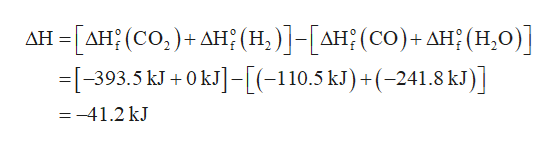# Using the standard enthalpies of formation, what is the standard enthalpy of reaction? CO(g)+H2O(g)⟶CO2(g)+H2(g)

Question
218 views

Using the standard enthalpies of formation, what is the standard enthalpy of reaction?

CO(g)+H2O(g)⟶CO2(g)+H2(g)
check_circle

Step 1

Enthalpy change of a reaction is calculated by subtracting the sum of enthalpies of formation of reactants from the sum of enthalpies of formation of products.

Step 2

The given reaction is,

CO(g) + H2O(g) → CO2(g) + H2(g)

The standard enthal...help_outlineImage Transcriptionclose-ΔΗ (CO, ) + ΔΗ; (Η. )] - [ΔΗ; (CO) + ΔΗ: (H0) ] -393.5 kJ +0kJ]-[(-110.5 kl)+(-241.8 kl)] ΔΗ =-41.2 kJ fullscreen

### Want to see the full answer?

See Solution

#### Want to see this answer and more?

Solutions are written by subject experts who are available 24/7. Questions are typically answered within 1 hour.*

See Solution
*Response times may vary by subject and question.
Tagged in

### General Chemistry